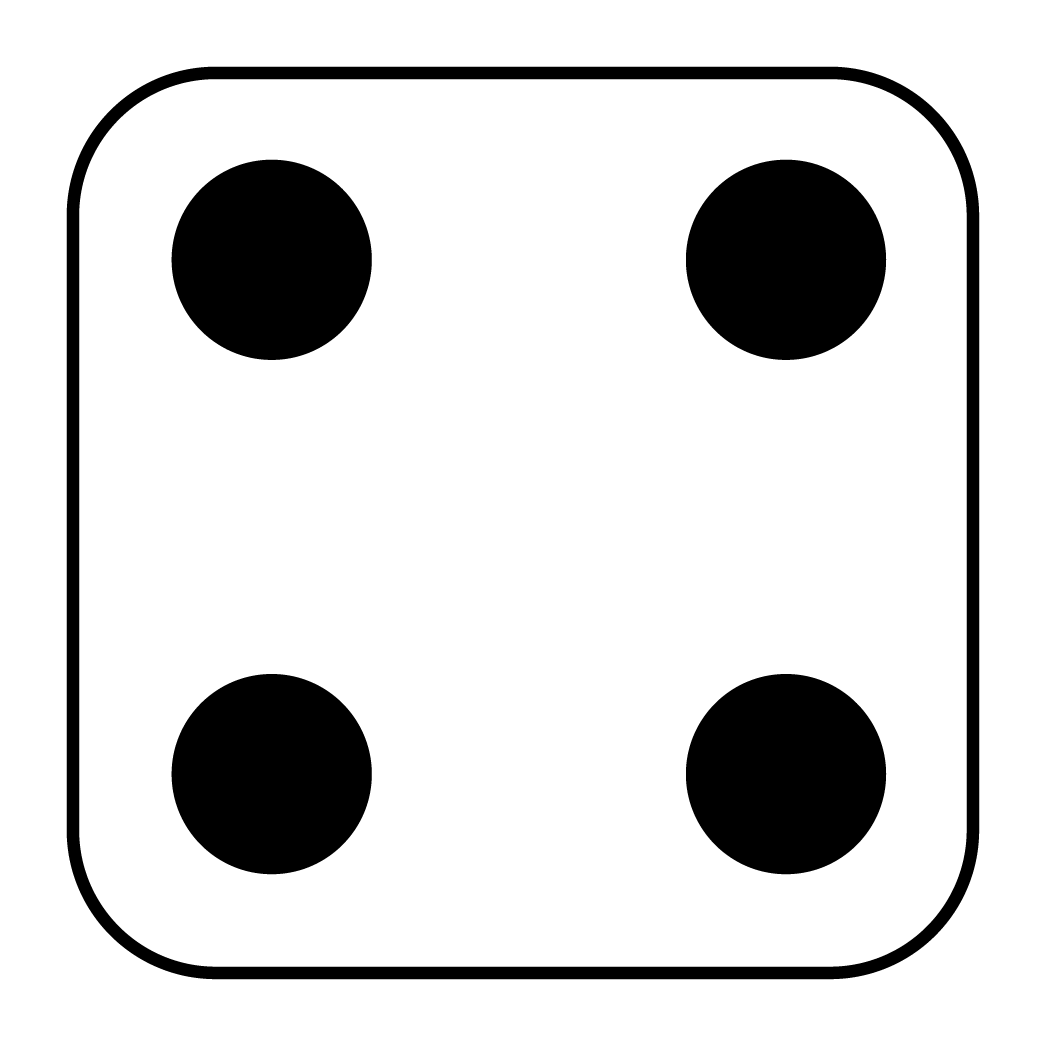## 16.2 Classical approach

What is the probability of rolling aon a die? The sample space has six possible outcomes (listed in Example 16.1) that are equally likely, and the event (‘rolling a’) comprises just one of those. Thus, \begin{align*} \text{Prob. of rolling a four} &= \frac{\text{The number of results that are a 4}}{\text{The number of possible results}}\\ &= \frac{1}{6}. \end{align*}

We can say that ‘the probability of rolling a 4 is 1/6,’ or ‘the probability of rolling ais 0.1667.’ The answer can also be expressed as a percentage (‘the probability of rolling ais 16.7%’).

The answer could also be interpreted as ‘the expected proportion of rolls that are ais 0.167.’ This approach to computing probabilities is called the classical approach to probability.

The chance of rolling ain the future is 0.167, but a roll of the die will either produce a, or will not produce a… and we don’t know which will occur.

Example 16.4 (Describing probability outcomes) Consider rolling a standard six-sided die again.

• The probability of rolling an even number is $$3 \div 6 = 0.5$$.
• The percentage of rolls that are expected to be even numbers is $$3 \div 6 \times 100 = 50$$%.
• The odds of rolling an even number is $$3\div 3 = 1$$.
Definition 16.4 (Classical approach to probability) In the classical approach to probability, the probability of an event occurring is the number of elements of the sample space included in the event, divided by the total number of elements in the sample space, when all outcomes are equally likely.

By this definition:

$\text{Prob. of an event} = \frac{\text{Number of equally-likely outcomes in the event of interest}}{\text{Total number of equally-likely outcomes}}$

Example 16.5 (Simple events) What is the probability of rolling a 2 on a die? What are the odds of rolling a 2 on a die?

Since the six possible outcomes in the sample space are equally likely:

$\text{Prob. of rolling a two} = \frac{\text{One outcome is a 2}}{\text{Six equally-likely outcomes}}.$ So the probability is $$\frac{1}{6} = 0.1667$$, or about 16.7%. Also, since the six possible outcomes are equally likely:

$\text{Odds of rolling a two} = \frac{\text{One outcomes is a two}}{\text{Five of the possible outcomes are not a two}}.$ So the odds of rolling a two is $$\frac{1}{5} = 0.2$$.

Example 16.6 (More complicated events) Consider rolling a standard six-sided die. There are six equally likely outcomes (Example 16.1) each with probability $$1/6$$ (or 16.7%) of occurring. The probability of rolling a 1 or a 2 is $$2/6$$ (or 33.3%).

Probabilities describe the likelihood that an event will occur before the outcome is known.

Odds and proportions can be used either before or after the outcome is known, provided the wording is correct. For example:

• Proportions describe how often an event has occurred after the outcome is known.
• Expected proportions describe the likelihood that an event will occur before the outcome is known.
The following example may help also.

Example 16.7 (Probabilities, proportions, odds) Before a fair coin is tossed:

• The probability of throwing a head is $$1/2 = 0.5$$.
• The expected proportion of heads in many coin tosses is 0.5.
• The odds of throwing a head is $$1/1 = 1$$.

If we have already tossed a coin 100 times and found 47 heads:

• The proportion of heads is $$47/100 = 0.47$$.
• The odds that we threw a head is $$47/53 = 0.887$$.
It makes no sense to talk about the ‘probability that we just threw a head,’ because the event has already occurred.